# How To Solve Cube Quickly

## How To Solve Cube Questions Quickly:-

Here in this Page How to Solve Cube Questions Quickly is given.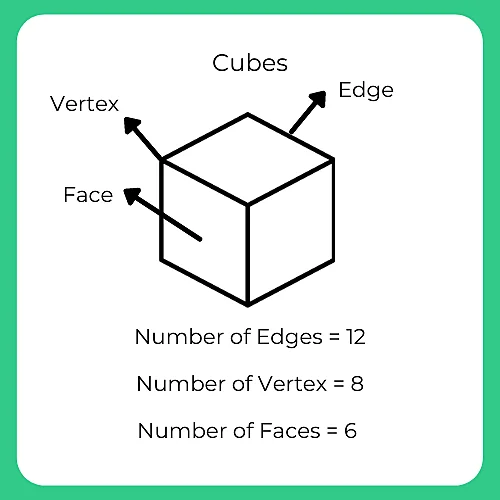### Solve Quickly Cube Questions on : Alphabet & Number Cubes:

Question 1: How many cubes can be formed from the given open image?

A. 1 and 2
B. 1,2,3 & 4
C. Only 3
D. Only 4

Explanation: As we see in the picture above, 5 is opposite to 6, 1 is opposite to 3, and 4 is opposite to 2, so 5 & 6, 1 & 3 and 4 and 6 cannot be together. Only option C follows this rule hence, it is correct.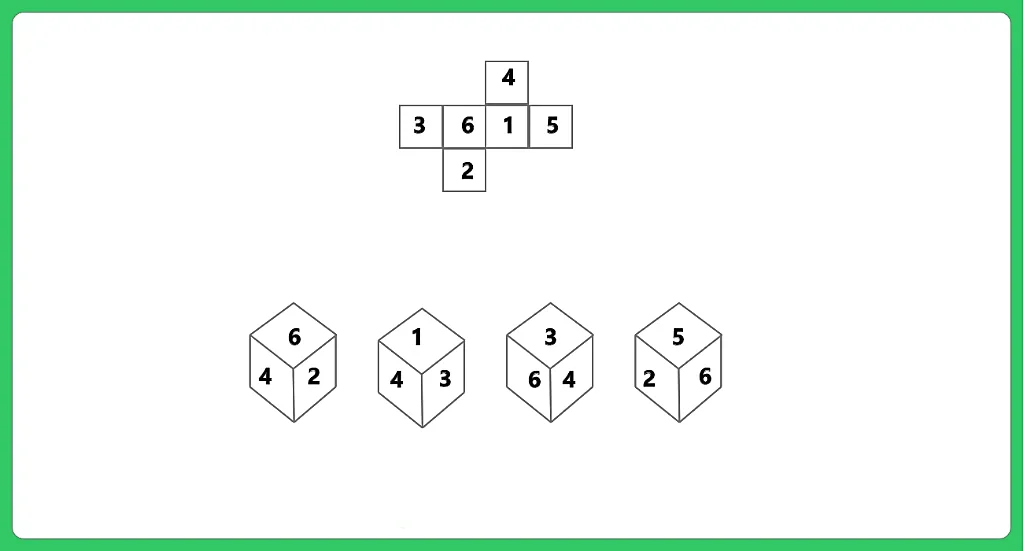Question 2. Which digit will come opposite to face 2 if the below given figure is folded?

A. 1
B. 4
C. 6
D. 2

Explanation: As per the rules to solve the cube if we want to know the opposite to the face 2, then first we need to consider it as a base. Hence its opposite will be then 1 only.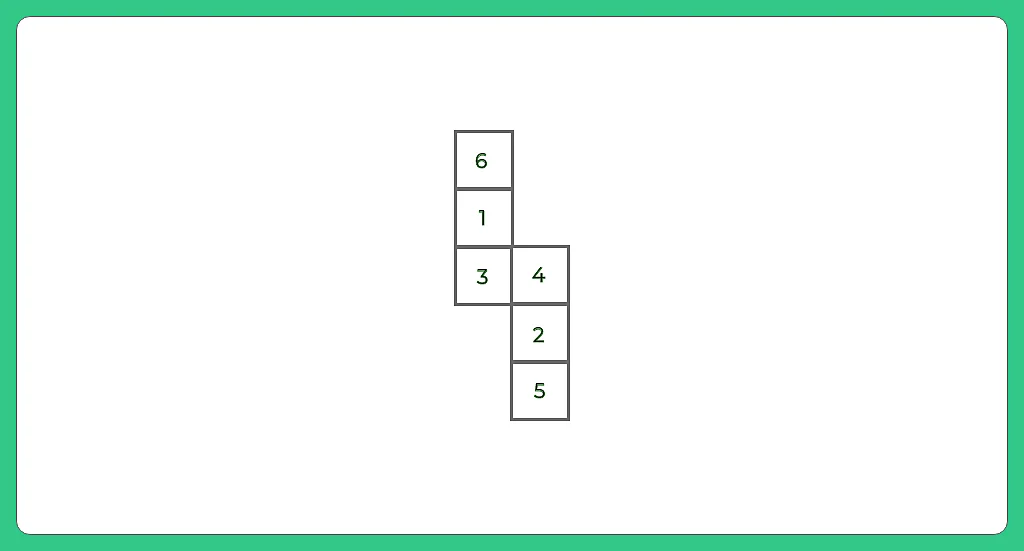### How To Solve Quickly Cube Question: Position of The CUBE

Question 1.

Which option is correct if we fold a piece of the paper to make a cube?

A. 2 only
B. 3 only
C. 4 only
D. 1, 2, and 3

Explanation: As per the rules of cube, we know that if we fold the main image then F will be opposite to B, E will be opposite to C and A will be opposite to D. now looking to the option we can simply eliminate image 1, 3 and 4 because F and B, E and C and A and D cannot be together. Hence only image 2 is accurate.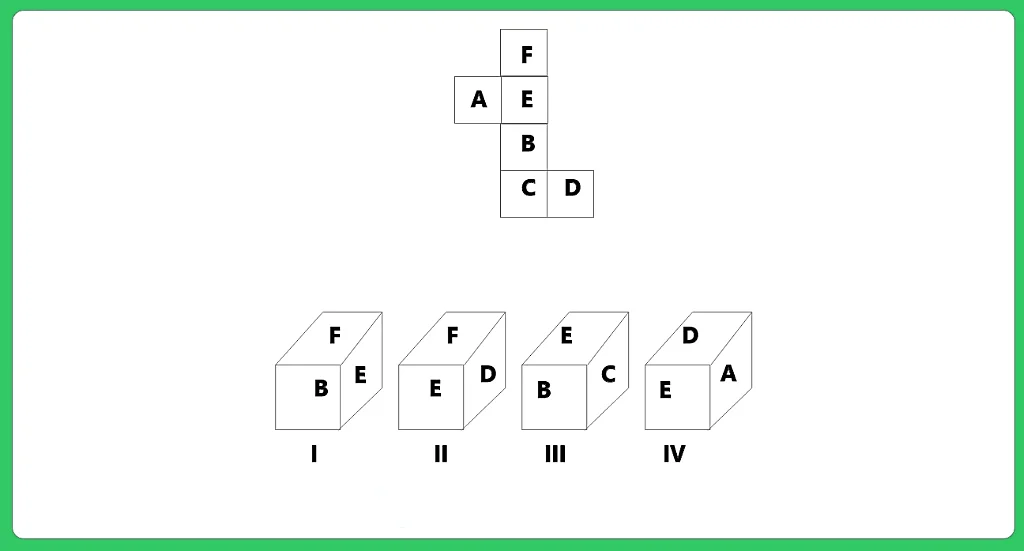Question 2:  Select an option which is alike to the main box given?

1. A
2. B
3. C
4. D

Explanation: As per the rules of cube of we fold main image, then K will be opposite to the triangle. The question mark will be opposite to the first blank side and divide sign will come opposite to another blank side. Option A and D does not follow this rule as in that both k and triangle are together. Further option C is also not possible because in that we need to exchanges the face position of cubes. Therefore only option B is accurate.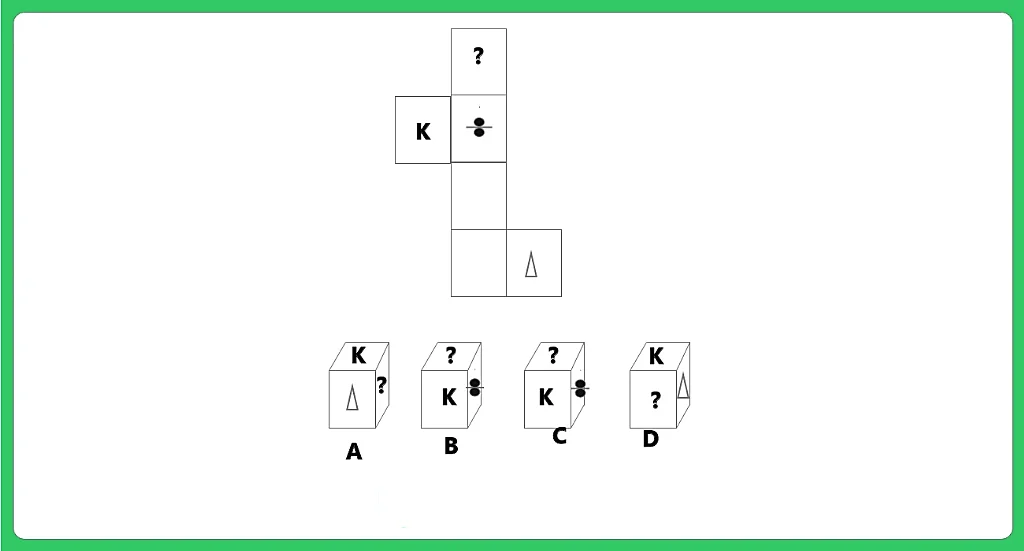### Related Banners

Get PrepInsta Prime & get Access to all 200+ courses offered by PrepInsta in One Subscription

## Get over 200+ course One Subscription

Courses like AI/ML, Cloud Computing, Ethical Hacking, C, C++, Java, Python, DSA (All Languages), Competitive Coding (All Languages), TCS, Infosys, Wipro, Amazon, DBMS, SQL and others